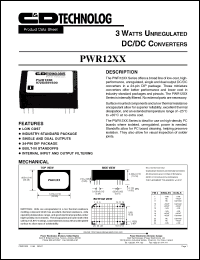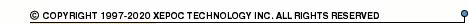More than4 307 195 components listedPWR12 series datasheets. Manufacturer: CANDD.

 PWR1200 3 watt unregulated DC/DC converter. Nom.input voltage 5VDC, rated output voltage 5VDC, rated output current 600mA. in 24-pin DIP package. Operational temperature range from -25°C to 85°C. Datasheet*) PWR1201 3 watt unregulated DC/DC converter. Nom.input voltage 5VDC, rated output voltage 12VDC, rated output current 250mA. in 24-pin DIP package. Operational temperature range from -25°C to 85°C. Datasheet*) PWR1202 3 watt unregulated DC/DC converter. Nom.input voltage 5VDC, rated output voltage 15VDC, rated output current 200mA. in 24-pin DIP package. Operational temperature range from -25°C to 85°C. Datasheet*) PWR1203 3 watt unregulated DC/DC converter. Nom.input voltage 5VDC, rated output voltage +-5VDC, rated output current +-300mA. in 24-pin DIP package. Operational temperature range from -25°C to 85°C. Datasheet*) PWR1204 3 watt unregulated DC/DC converter. Nom.input voltage 5VDC, rated output voltage +-12VDC, rated output current +-125mA. in 24-pin DIP package. Operational temperature range from -25°C to 85°C. Datasheet*) PWR1205 3 watt unregulated DC/DC converter. Nom.input voltage 5VDC, rated output voltage +-15VDC, rated output current +-100mA. in 24-pin DIP package. Operational temperature range from -25°C to 85°C. Datasheet*) PWR1206 3 watt unregulated DC/DC converter. Nom.input voltage 12VDC, rated output voltage 5VDC, rated output current 600mA. in 24-pin DIP package. Operational temperature range from -25°C to 85°C. Datasheet*) PWR1207 3 watt unregulated DC/DC converter. Nom.input voltage 12VDC, rated output voltage 12VDC, rated output current 250mA. in 24-pin DIP package. Operational temperature range from -25°C to 85°C. Datasheet*) PWR1208 3 watt unregulated DC/DC converter. Nom.input voltage 12VDC, rated output voltage 15VDC, rated output current 200mA. in 24-pin DIP package. Operational temperature range from -25°C to 85°C. Datasheet*) PWR1209 3 watt unregulated DC/DC converter. Nom.input voltage 12VDC, rated output voltage +-5VDC, rated output current +-300mA. in 24-pin DIP package. Operational temperature range from -25°C to 85°C. Datasheet*) PWR1210 3 watt unregulated DC/DC converter. Nom.input voltage 12VDC, rated output voltage +-12VDC, rated output current +-125mA. in 24-pin DIP package. Operational temperature range from -25°C to 85°C. Datasheet*) PWR1211 3 watt unregulated DC/DC converter. Nom.input voltage 12VDC, rated output voltage +-15VDC, rated output current +-100mA. in 24-pin DIP package. Operational temperature range from -25°C to 85°C. Datasheet*) PWR1212 3 watt unregulated DC/DC converter. Nom.input voltage 15VDC, rated output voltage 5VDC, rated output current 600mA. in 24-pin DIP package. Operational temperature range from -25°C to 85°C. Datasheet*) PWR1213 3 watt unregulated DC/DC converter. Nom.input voltage 15VDC, rated output voltage 12VDC, rated output current 250mA. in 24-pin DIP package. Operational temperature range from -25°C to 85°C. Datasheet*) PWR1214 3 watt unregulated DC/DC converter. Nom.input voltage 15VDC, rated output voltage 15VDC, rated output current 200mA. in 24-pin DIP package. Operational temperature range from -25°C to 85°C. Datasheet*) PWR1215 3 watt unregulated DC/DC converter. Nom.input voltage 15VDC, rated output voltage +-5VDC, rated output current +-300mA. in 24-pin DIP package. Operational temperature range from -25°C to 85°C. Datasheet*) PWR1216 3 watt unregulated DC/DC converter. Nom.input voltage 15VDC, rated output voltage +-12VDC, rated output current +-125mA. in 24-pin DIP package. Operational temperature range from -25°C to 85°C. Datasheet*) PWR1217 3 watt unregulated DC/DC converter. Nom.input voltage 15VDC, rated output voltage +-15VDC, rated output current +-100mA. in 24-pin DIP package. Operational temperature range from -25°C to 85°C. Datasheet*) PWR1218 3 watt unregulated DC/DC converter. Nom.input voltage 24VDC, rated output voltage 5VDC, rated output current 600mA. in 24-pin DIP package. Operational temperature range from -25°C to 85°C. Datasheet*) PWR1219 3 watt unregulated DC/DC converter. Nom.input voltage 24VDC, rated output voltage 12VDC, rated output current 250mA. in 24-pin DIP package. Operational temperature range from -25°C to 85°C. Datasheet*) PWR1220 3 watt unregulated DC/DC converter. Nom.input voltage 24VDC, rated output voltage 15VDC, rated output current 200mA. in 24-pin DIP package. Operational temperature range from -25°C to 85°C. Datasheet*) PWR1221 3 watt unregulated DC/DC converter. Nom.input voltage 24VDC, rated output voltage +-5VDC, rated output current +-300mA. in 24-pin DIP package. Operational temperature range from -25°C to 85°C. Datasheet*) PWR1222 3 watt unregulated DC/DC converter. Nom.input voltage 24VDC, rated output voltage +-12VDC, rated output current +-125mA. in 24-pin DIP package. Operational temperature range from -25°C to 85°C. Datasheet*) PWR1223 3 watt unregulated DC/DC converter. Nom.input voltage 24VDC, rated output voltage +-15VDC, rated output current +-100mA. in 24-pin DIP package. Operational temperature range from -25°C to 85°C. Datasheet*) PWR1240 3 watt unregulated DC/DC converter. Nom.input voltage 5VDC, rated output voltage 9VDC, rated output current 333mA. in 24-pin DIP package. Operational temperature range from -25°C to 85°C. Datasheet*) PWR1241 3 watt unregulated DC/DC converter. Nom.input voltage 12VDC, rated output voltage 9VDC, rated output current 333mA. in 24-pin DIP package. Operational temperature range from -25°C to 85°C. Datasheet*) PWR1242 3 watt unregulated DC/DC converter. Nom.input voltage 15VDC, rated output voltage 9VDC, rated output current 333mA. in 24-pin DIP package. Operational temperature range from -25°C to 85°C. Datasheet*) PWR1243 3 watt unregulated DC/DC converter. Nom.input voltage 24VDC, rated output voltage 9VDC, rated output current 333mA. in 24-pin DIP package. Operational temperature range from -25°C to 85°C. Datasheet*) PWR12XX 3 WATT UNREGULATED DC/DC CONVERTERS Datasheet*)